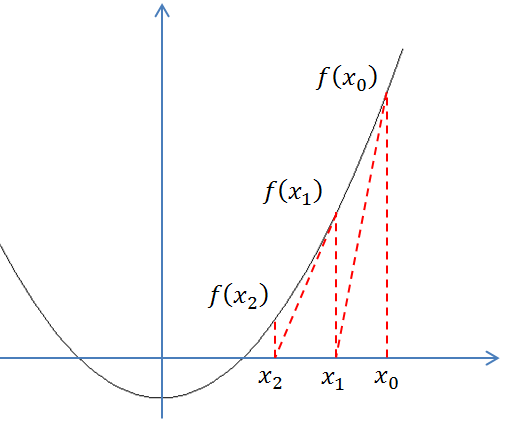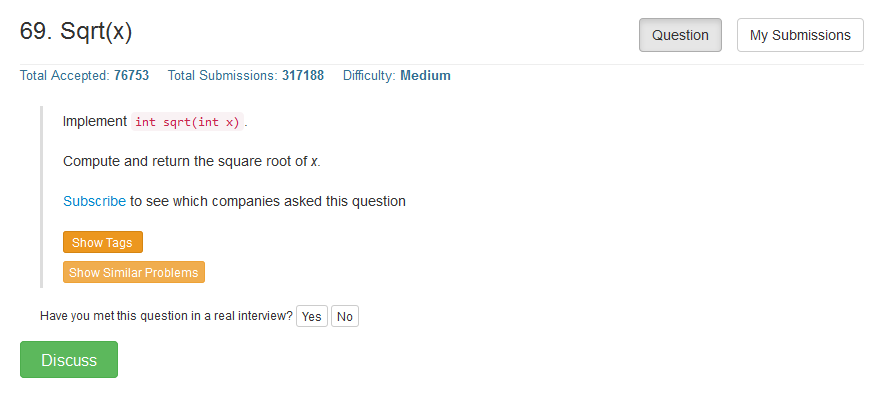# 牛顿迭代法与一道经典编程问题

牛顿迭代法（Newton’s method）又称为牛顿-拉夫逊（拉弗森）方法（Newton-Raphson method），它是牛顿在17世纪提出的一种在实数域和复数域上近似求解方程的方法。既然牛顿迭代法可以用来求解方程的根，那么不妨以方程 x2=n$x^2=n$ 为例，来试着求解它的根。为此。令f(x)=x2n$f(x) = x^2 - n$， 也就是相当于求解 f(x)=0$f(x) = 0$ 的解，如上图所示。

首先随便找一个初始值 x0$x_0$，如果 x0$x_0$不是解，做一个经过 (x0,f(x0))$( x_0,f( x_0))$ 这个点的切线，与x$x$轴的交点为x1$x_1$。同样的道理，如果 x1$x_1$不是解，做一个经过(x1,f(x1))$( x_1,f( x_1))$这个点的切线，与x$x$轴的交点为x2$x_2$。 以此类推。以这样的方式得到的xi$x_i$会无限趋近于 f(x)=0$f(x) = 0$ 的解。

f(x)=f(xi)+f(xi)(xxi)

xi+1=xif(xi)f(xi)

xi+1=xix2in2xi=xixi2+n2xi=xi2+n2xi

Leetcode上也有一道经典面试题目涉及到开平方函数的实现，如下class Solution {
public:
int mySqrt(int x) {
if (x ==0)
return 0;
double pre;
double cur = 1;
do
{
pre = cur;
cur = x / (2 * pre) + pre / 2.0;
} while (abs(cur - pre) > 0.00001);
return int(cur);
}
};©️2019 CSDN 皮肤主题: 精致技术 设计师: CSDN官方博客